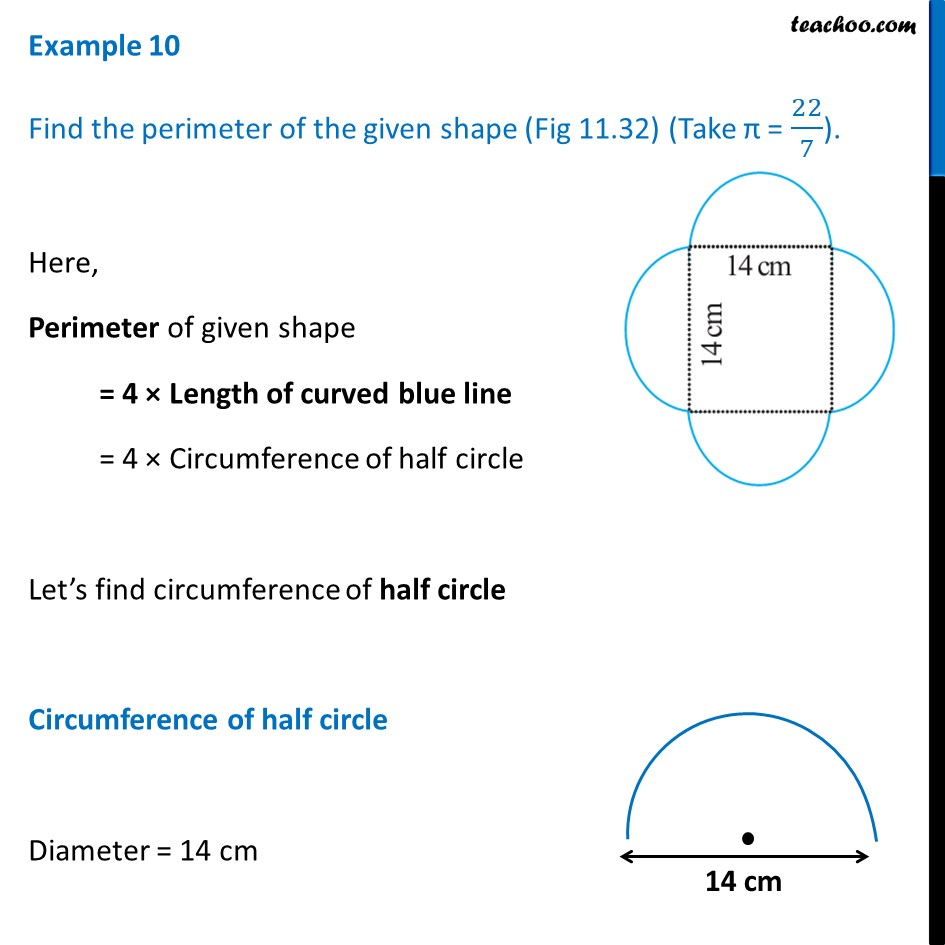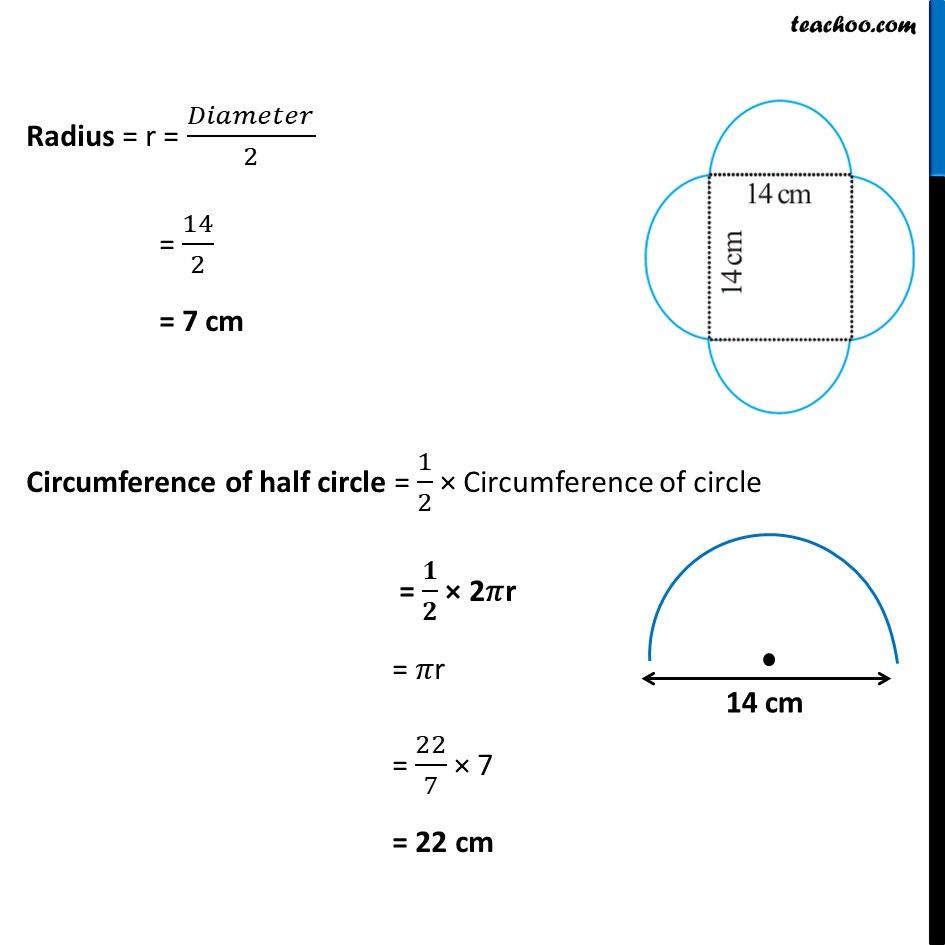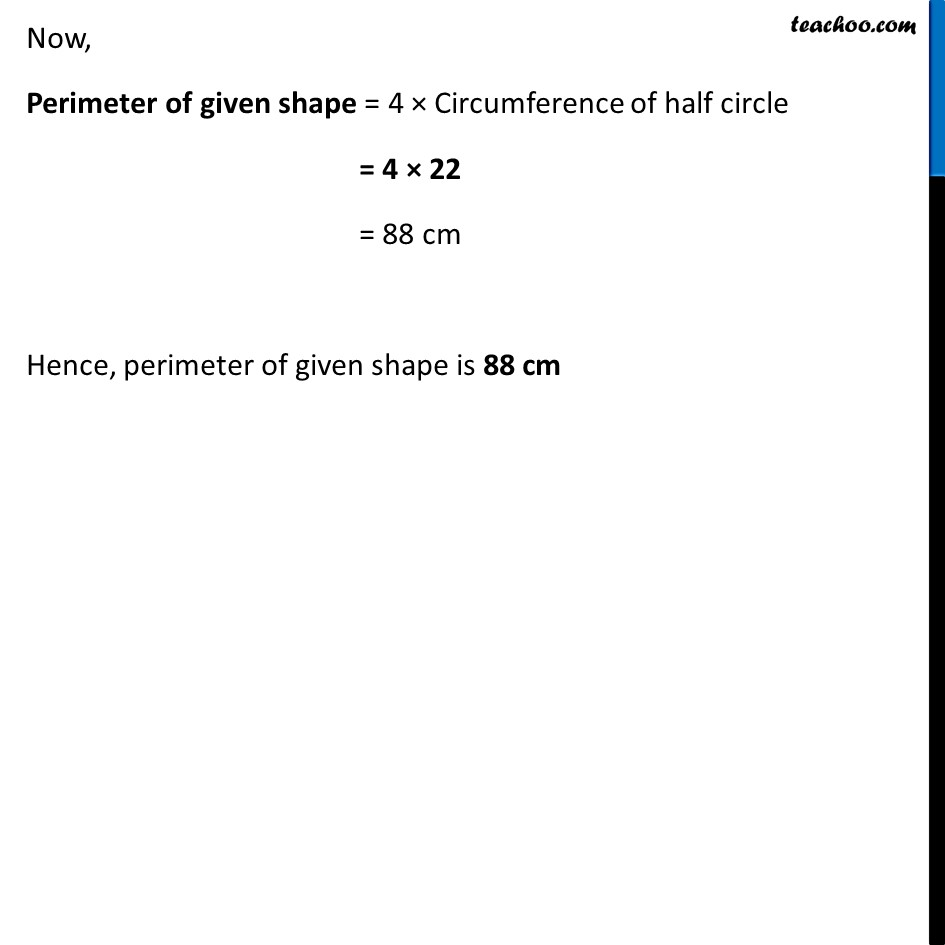Examples

Chapter 9 Class 7 Perimeter and Area
Serial order wiseLearn in your speed, with individual attention - Teachoo Maths 1-on-1 Class

### Transcript

Example 10 Find the perimeter of the given shape (Fig 11.32) (Take π = 22/7).Here, Perimeter of given shape = 4 × Length of curved blue line = 4 × Circumference of half circle Let’s find circumference of half circle Circumference of half circle Diameter = 14 cm Radius = r = 𝐷𝑖𝑎𝑚𝑒𝑡𝑒𝑟/2 = 14/2 = 7 cm Circumference of half circle = 1/2 × Circumference of circle = 𝟏/𝟐 × 2𝜋r = 𝜋r = 22/7 × 7 = 22 cm Now, Perimeter of given shape = 4 × Circumference of half circle = 4 × 22 = 88 cm Hence, perimeter of given shape is 88 cm